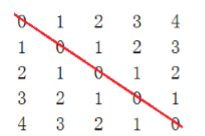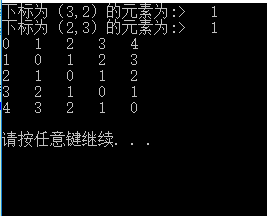20 篇文章 0 订阅

# 矩阵

### 三、什么是特殊矩阵？

1.特殊矩阵是矩阵；
2.矩阵中的元素有许多值相同或者有许多零元素；
3.矩阵中值相同的元素或零元素，它们的分布是有一定规律的；
ps：这三个条件缺一不可

# 对称矩阵

### 一、什么是对称矩阵？

1.对称矩阵是矩阵的一种存在形式
2.设一个N*N的矩阵A，A中任意元素A(i,j),当且仅当A(i,j)=A(j,i)（0 <=i <=N-1&&
0 <=j <=N-1），则矩阵A是对称矩阵ps：以矩阵的对角线为分割，分为上三角和下三角

### 二、对称矩阵的特性？

1.对称矩阵是一个方阵，即就是行和列长度相等
2.对称矩阵中的所有元素都是相互对称的，即就是矩阵的下三角和上三角是对称的

### 三、对阵矩阵中元素的位置

1.行=列时，此时表明此元素位于对角线
2.行>列时，表明此元素位于下三角中
3.行<列时，表明此元素位于上三角中

### 四、对称矩阵的存储

    在存储下三角时，可以使用一个二维数组来保存矩阵中的数据，但是这样的

存储矩阵下三角元素个数等于(（1+N)N)/2，所以数组的空间大小就

for (size_t i = 0; i < N; ++i) { //存储行
for (size_t j = 0; j <= i; ++j) { //存储列
_array[index++] = array[(i*N) + j];

### 五、对称矩阵的访问

    由于在存储时，只存储了一个下三角，但是对阵矩阵的访问必须可以访问整

T &Acess(size_t row, size_t col) {
if (row < col)
swap(row, col);
return _array[(((row + 1)*row) >> 1) + col];
}

### 源代码

#include <iostream>
#include <vector>
using namespace std;
template<class T>
class SymmetricMatrix {
public:
SymmetricMatrix(const T *array, size_t N)
:_row(N)
, _col(N) {
size_t index = 0;//是一维数组的下标
_array.resize(((1 + N) * N) >> 1); //开辟空间，矩阵下三角元素共有(（1 + N)N) / 2
for (size_t i = 0; i < N; ++i) { //存储行
for (size_t j = 0; j <= i; ++j) { //存储列
_array[index++] = array[(i*N) + j];//二维数组在内存中，其实也是按一位数组的形式存储着的
}
}
}
T &Acess(size_t row, size_t col) {
if (row < col)//如果是要访问上三角的元素，那就把需要访问的元素对称到下三角中，然后访问下三角
swap(row, col);
return _array[(((row + 1)*row) >> 1) + col];
}
void Print() { //打印矩阵
for (size_t i = 0; i < _row; ++i) {
for (size_t j = 0; j < _col; ++j)
cout << Acess(i, j);
cout << endl;
}
cout << endl;
}
private:
vector<T> _array;//用来存储矩阵下三角的一维数组
size_t _row;//矩阵的行
size_t _col;//矩阵的列

};
void TestSymmetricMatrix() {
int array = {
{ 0, 1, 2, 3, 4 },
{ 1, 0, 1, 2, 3 },
{ 2, 1, 0, 1, 2 },
{ 3, 2, 1, 0, 1 },
{ 4, 3, 2, 1, 0 }
};
SymmetricMatrix<int>  sm((int *)array, sizeof(array) / sizeof(array));
//因为array是二维数组，而构造函数的参数是一维数组，所以需要把二维的数组array强转为一位数组
//注意二维数组名代表的是二维数组首元素的地址，只是此处说的首元素是二维数组首行
cout << "下标为（3,2）的元素为:>   " << sm.Acess(3, 2) << endl;
cout << "下标为（2,3）的元素为:>   " << sm.Acess(2, 3) << endl;
sm.Print();
cout << endl;
}
int main() {
TestSymmetricMatrix();
system("pause");
return 0;
}04-16148709-281899
01-066785
04-253609
07-011051
04-193456
05-091914
10-01
11-262383
04-08295
05-30
05-312138
03-1668
08-05646
03-261万+点击重新获取扫码支付余额充值